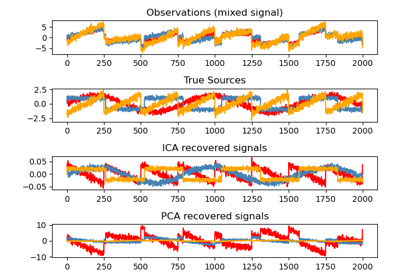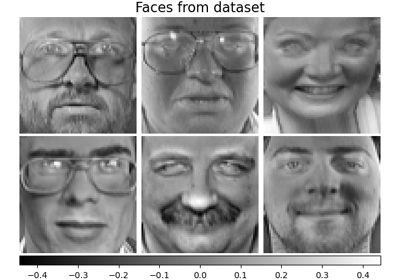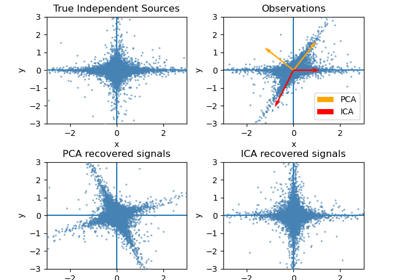# sklearn.decomposition.FastICA¶

class sklearn.decomposition.FastICA(n_components=None, *, algorithm='parallel', whiten='warn', fun='logcosh', fun_args=None, max_iter=200, tol=0.0001, w_init=None, random_state=None)[source]

FastICA: a fast algorithm for Independent Component Analysis.

The implementation is based on .

Read more in the User Guide.

Parameters:
n_componentsint, default=None

Number of components to use. If None is passed, all are used.

algorithm{‘parallel’, ‘deflation’}, default=’parallel’

Specify which algorithm to use for FastICA.

whitenstr or bool, default=”warn”

Specify the whitening strategy to use.

• If ‘arbitrary-variance’ (default), a whitening with variance arbitrary is used.

• If ‘unit-variance’, the whitening matrix is rescaled to ensure that each recovered source has unit variance.

• If False, the data is already considered to be whitened, and no whitening is performed.

Deprecated since version 1.1: Starting in v1.3, whiten='unit-variance' will be used by default. whiten=True is deprecated from 1.1 and will raise ValueError in 1.3. Use whiten=arbitrary-variance instead.

fun{‘logcosh’, ‘exp’, ‘cube’} or callable, default=’logcosh’

The functional form of the G function used in the approximation to neg-entropy. Could be either ‘logcosh’, ‘exp’, or ‘cube’. You can also provide your own function. It should return a tuple containing the value of the function, and of its derivative, in the point. The derivative should be averaged along its last dimension. Example:

def my_g(x):
return x ** 3, (3 * x ** 2).mean(axis=-1)

fun_argsdict, default=None

Arguments to send to the functional form. If empty or None and if fun=’logcosh’, fun_args will take value {‘alpha’ : 1.0}.

max_iterint, default=200

Maximum number of iterations during fit.

tolfloat, default=1e-4

A positive scalar giving the tolerance at which the un-mixing matrix is considered to have converged.

w_initndarray of shape (n_components, n_components), default=None

Initial un-mixing array. If w_init=None, then an array of values drawn from a normal distribution is used.

random_stateint, RandomState instance or None, default=None

Used to initialize w_init when not specified, with a normal distribution. Pass an int, for reproducible results across multiple function calls. See Glossary.

Attributes:
components_ndarray of shape (n_components, n_features)

The linear operator to apply to the data to get the independent sources. This is equal to the unmixing matrix when whiten is False, and equal to np.dot(unmixing_matrix, self.whitening_) when whiten is True.

mixing_ndarray of shape (n_features, n_components)

The pseudo-inverse of components_. It is the linear operator that maps independent sources to the data.

mean_ndarray of shape(n_features,)

The mean over features. Only set if self.whiten is True.

n_features_in_int

Number of features seen during fit.

New in version 0.24.

feature_names_in_ndarray of shape (n_features_in_,)

Names of features seen during fit. Defined only when X has feature names that are all strings.

New in version 1.0.

n_iter_int

If the algorithm is “deflation”, n_iter is the maximum number of iterations run across all components. Else they are just the number of iterations taken to converge.

whitening_ndarray of shape (n_components, n_features)

Only set if whiten is ‘True’. This is the pre-whitening matrix that projects data onto the first n_components principal components.

PCA

Principal component analysis (PCA).

IncrementalPCA

Incremental principal components analysis (IPCA).

KernelPCA

Kernel Principal component analysis (KPCA).

MiniBatchSparsePCA

Mini-batch Sparse Principal Components Analysis.

SparsePCA

Sparse Principal Components Analysis (SparsePCA).

References



A. Hyvarinen and E. Oja, Independent Component Analysis: Algorithms and Applications, Neural Networks, 13(4-5), 2000, pp. 411-430.

Examples

>>> from sklearn.datasets import load_digits
>>> from sklearn.decomposition import FastICA
>>> transformer = FastICA(n_components=7,
...         random_state=0,
...         whiten='unit-variance')
>>> X_transformed = transformer.fit_transform(X)
>>> X_transformed.shape
(1797, 7)


Methods

 fit(X[, y]) Fit the model to X. fit_transform(X[, y]) Fit the model and recover the sources from X. get_feature_names_out([input_features]) Get output feature names for transformation. get_params([deep]) Get parameters for this estimator. inverse_transform(X[, copy]) Transform the sources back to the mixed data (apply mixing matrix). set_params(**params) Set the parameters of this estimator. transform(X[, copy]) Recover the sources from X (apply the unmixing matrix).
fit(X, y=None)[source]

Fit the model to X.

Parameters:
Xarray-like of shape (n_samples, n_features)

Training data, where n_samples is the number of samples and n_features is the number of features.

yIgnored

Not used, present for API consistency by convention.

Returns:
selfobject

Returns the instance itself.

fit_transform(X, y=None)[source]

Fit the model and recover the sources from X.

Parameters:
Xarray-like of shape (n_samples, n_features)

Training data, where n_samples is the number of samples and n_features is the number of features.

yIgnored

Not used, present for API consistency by convention.

Returns:
X_newndarray of shape (n_samples, n_components)

Estimated sources obtained by transforming the data with the estimated unmixing matrix.

get_feature_names_out(input_features=None)[source]

Get output feature names for transformation.

Parameters:
input_featuresarray-like of str or None, default=None

Only used to validate feature names with the names seen in fit.

Returns:
feature_names_outndarray of str objects

Transformed feature names.

get_params(deep=True)[source]

Get parameters for this estimator.

Parameters:
deepbool, default=True

If True, will return the parameters for this estimator and contained subobjects that are estimators.

Returns:
paramsdict

Parameter names mapped to their values.

inverse_transform(X, copy=True)[source]

Transform the sources back to the mixed data (apply mixing matrix).

Parameters:
Xarray-like of shape (n_samples, n_components)

Sources, where n_samples is the number of samples and n_components is the number of components.

copybool, default=True

If False, data passed to fit are overwritten. Defaults to True.

Returns:
X_newndarray of shape (n_samples, n_features)

Reconstructed data obtained with the mixing matrix.

set_params(**params)[source]

Set the parameters of this estimator.

The method works on simple estimators as well as on nested objects (such as Pipeline). The latter have parameters of the form <component>__<parameter> so that it’s possible to update each component of a nested object.

Parameters:
**paramsdict

Estimator parameters.

Returns:
selfestimator instance

Estimator instance.

transform(X, copy=True)[source]

Recover the sources from X (apply the unmixing matrix).

Parameters:
Xarray-like of shape (n_samples, n_features)

Data to transform, where n_samples is the number of samples and n_features is the number of features.

copybool, default=True

If False, data passed to fit can be overwritten. Defaults to True.

Returns:
X_newndarray of shape (n_samples, n_components)

Estimated sources obtained by transforming the data with the estimated unmixing matrix.

## Examples using sklearn.decomposition.FastICA¶Blind source separation using FastICA

Blind source separation using FastICAFaces dataset decompositions

Faces dataset decompositionsFastICA on 2D point clouds

FastICA on 2D point clouds